# Irrational Partitions

This section is discussing the 200 residual objects that goes irrational along the pattern within the 4th dimension from the 2nd to 3rd prime identity in regards to the three (3) zones where each represents the basic arithmetic operations of Euler's identity.

``````---+-----+-----
1 | {1} | {9}
---+-----+-----
2 | 10  |{32}
---+-----+-----
3 | 33  | 63
---+-----+-----
4 |{64} | 101
---+-----+-----
5 |{102}| 120
---+-----+-----
6 | 121 |{189}
---+-----+-----
7 | 190 |{200}
---+-----+-----
``````

There are some mathematical shape of this residual objects. Torus is basically a donut shape, which has the property of of having variable Gaussian curvature. Some parts of the surface has positive curvature, others zero, others negative.

The blue parts of the torus above have positive curvature, the red parts negative and the top grey band has zero curvature. If our 3 dimensional space was like the surface areas of a 4 dimensional torus, the parts would have different angle sums.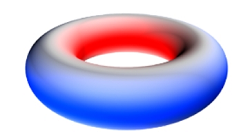These are two more bizarre shapes with strange properties. Mobius strip only has one side, if you start anywhere on its surface and follow the curvature round you will eventually return to the same place having travelled on every part of the surface.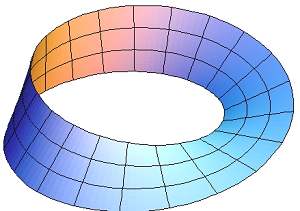The Klein bottle is in someways a 3D version of the Mobius strip and even though it exists in 3 dimensions, to make a true one you need to "fold through" the 4th dimension.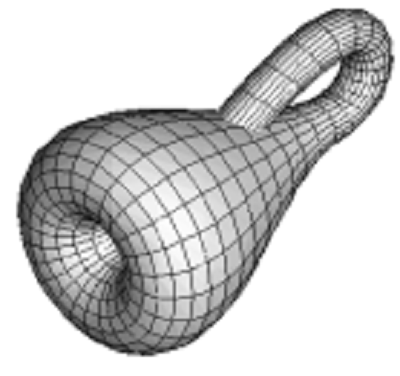The torus is actually one of the current theories of the shape of universe while the klein bottle is shape of the idea in theoretical physics called string theory that reality is made up of infinitesimal vibrating strings, smaller than atoms, electrons or quarks

Finally, there exist scenarios in which there could actually be more than 4D of spacetime. String theories require extra dimensions of spacetime for their mathematical consistency. In string theory, spacetime is 26-dimensional, while in superstring theory it is 10-dimensional, and in M-theory it is 11-dimensional (Wikipedia).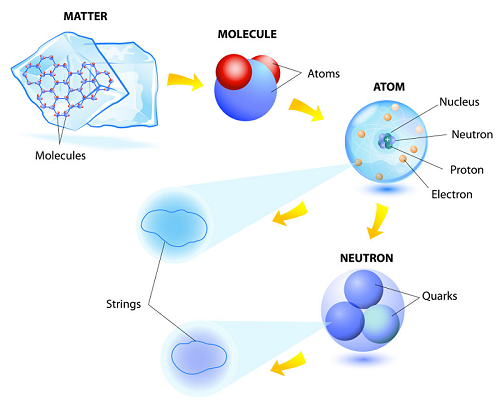These are situations where theories in two or three spacetime dimensions are no more useful. This classification theorem identifies several infinite families of groups as well as 26 additional groups which do not fit into any family.

## The three (3) zones

In order to describe real physical phenomena using string theory, one must therefore imagine scenarios in which these extra dimensions would not be observed in experiments so it would become the irrational partitions.

[(6 + 6) x 6] + [6 + (6 x 6)] = 72 + 42 = 71 + 42 + 1 = 114 objects

``````layer | node | sub |  i  |  f
------+------+-----+----------
|      |     |  1  | -----------------------  71 = 72-1
|      |  1  +-----+                        |
|  1   |     |  2  | (5)                    |
|      |-----+-----+                        |
|      |     |  3  | ---------              |
1   +------+  2  +-----+----      |             |
|      |     |  4  |          5x ---        |
|      +-----+-----+          |     |       |
|  2   |     |  5  | (7) -----      |       |
|      |  3  +-----+                |       |
289+11=300   |     |  6  |                |       |
------+------+-----+-----+----- 72 x 6   7x --- 11x = 77 (rational)
|      |     |  7  |                |       |
|      |  4  +-----+                |       |
|  3   |     |  8  | (11)  ---      |       |
|      +-----+-----+          |     |       |
|      |     |  9  |          2x ---        |
2   +------|  5  +-----+-----     |             |
|      |     |  10 | ---------              |
|      |-----+-----+                        |
|  4   |     |  11 | (13) ------------------  71 = 72-1
|      |  6  +-----+
329+71=400   |     |  12 |------------------------  70 = 72-2
------+------+-----+-----+
|      |     |  13 |
|      |  7  +-----+
|  5   |     |  14 | (17) ◄---------------------------
|      |-----+-----+
|      |     |  15 | ◄-- 42 x 6 partitions of 13 (irrational)
3   +------+  8  +-----+-----
|      |     |  16 |      ◄---------------------------
|      |-----+-----+
|  6   |     |  17 | (19)
|      |  9  +-----+
168+32=200   |  |  |  18 |------------------------  68 = 72-4
------|------|--|--+-----+
900 -----
``````

This irrational parts took the finiteness position of middle zero axis = 15 on our 19 vs 18 Scenario contained in three (3) groups of five (5) of integer number partitions where each groups represents the three (3) basic arithmetic operations of Euler's identity.

329 + 109 + 109 + 71 = 329 + 289 = 618 = 1000/1.618 = 1000/φ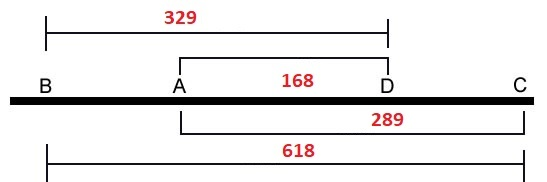The 200 objects that goes from the 2nd to 3rd prime identity would take position as multiplication zone by given of 100x2=200 which is then be used in turn to 100+2=102 (addition zone) and 100^2=10000 (exponentiation zone)

2 + 60 + 40 = 102

``````1st layer:
It has a total of 1000 numbers
Total primes = π(1000) = 168 primes

2nd layer:
It will start by π(168)+1 as the 40th prime
It has 100x100 numbers or π(π(10000)) = 201 primes
Total cum primes = 168 + (201-40) = 168+161 = 329 primes

3rd layer:
Behave the same as 2nd layer which has a total of 329 primes
The primes will start by π(π(π(1000th prime)))+1 as the 40th prime
This 1000 primes will become 1000 numbers by 1st layer of the next level
Total of all primes = 329 + (329-40) = 329+289 = 618 = 619-1 = 619 primes - Δ1
``````

The Schrödinger equation is a linear partial differential equation that governs the wave function of a quantum mechanics. It is a key result in quantum-mechanical system, and its discovery was a significant landmark in the development of the subject.

Complex plot of a wave function that satisfies the nonrelativistic Schrödinger equation with V = 0. In other words, this corresponds to a particle traveling freely through empty space (Wikipedia).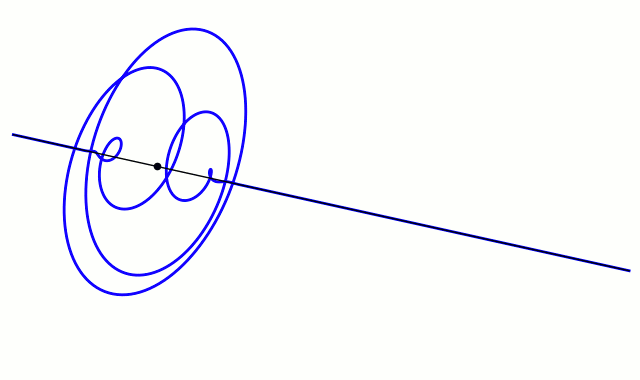## The modular function

Consequently, we only need to fold a 30's cycle as in the illustration 7 so that we can identify the opposite prime positions that form their specific pairs in a specific convolution.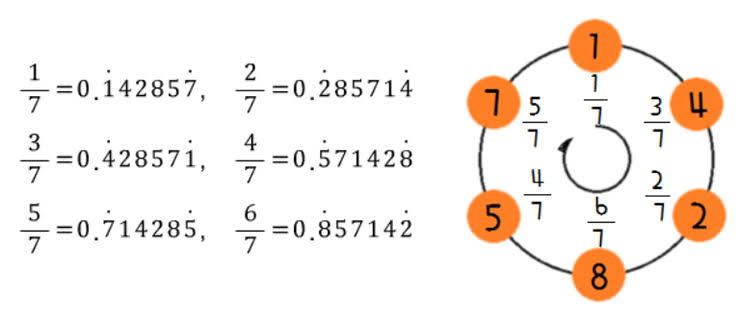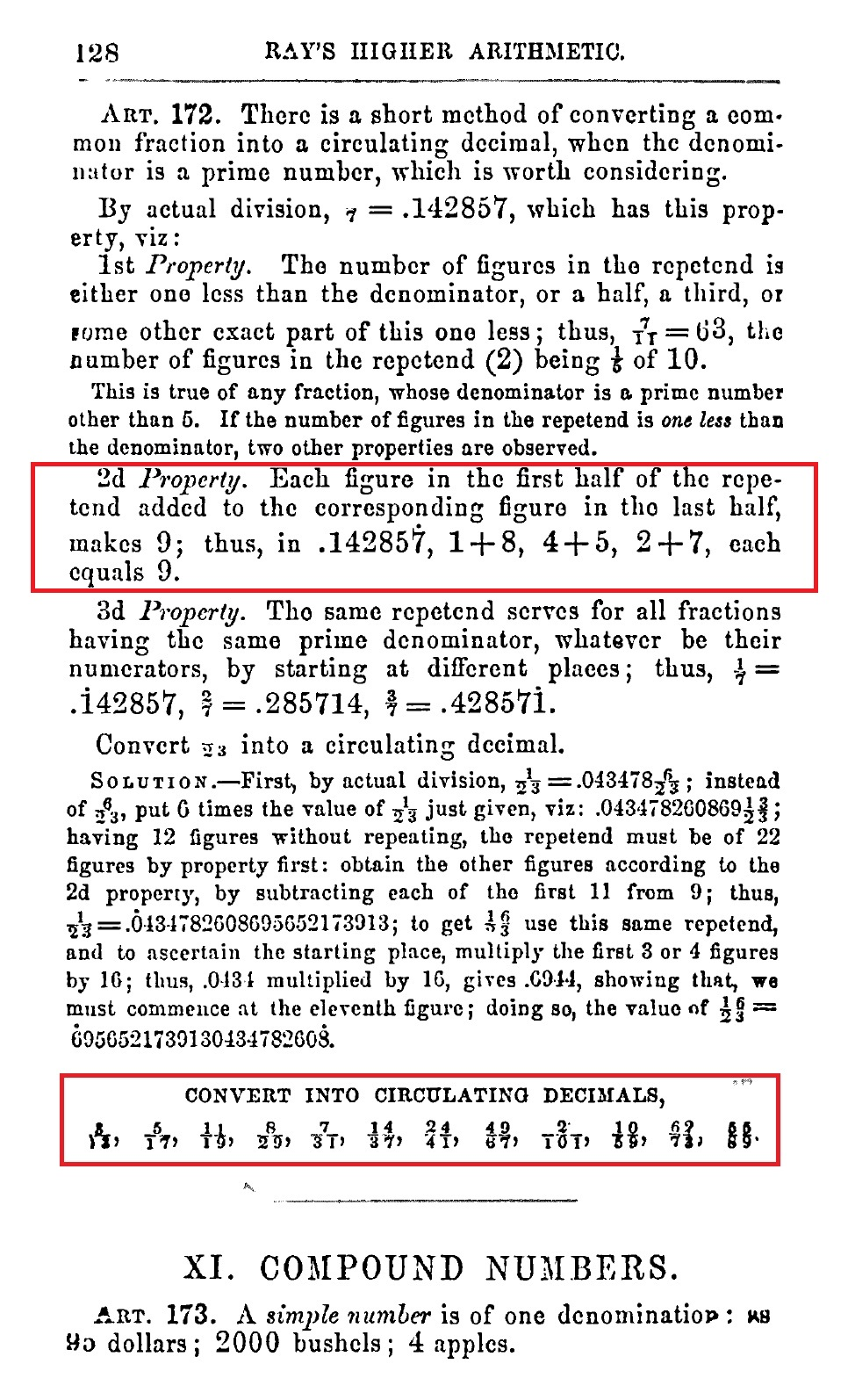These two (2) exponent are acting as the exchange zones of the MEC30 behaviour of the other two operators. This interactions is managed in between 4 identities of (26 to 29) and 2 identities (30 to 31) by means of Triangular waves.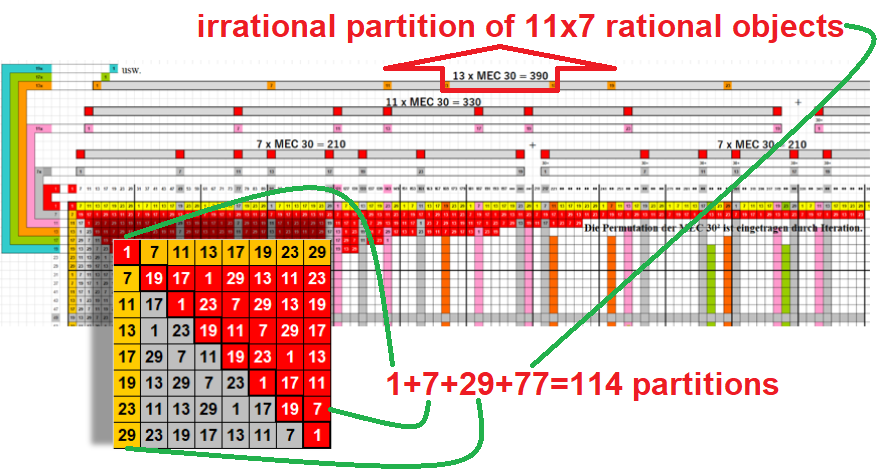A seemingly unrelated construction is the j-function of number theory. This object belongs to a special class of functions called modular functions, whose graphs form a certain kind of repeating pattern.

Although this function appears in a branch of mathematics that seems very different from the theory of finite groups, the two subjects turn out to be intimately related (Wikipedia).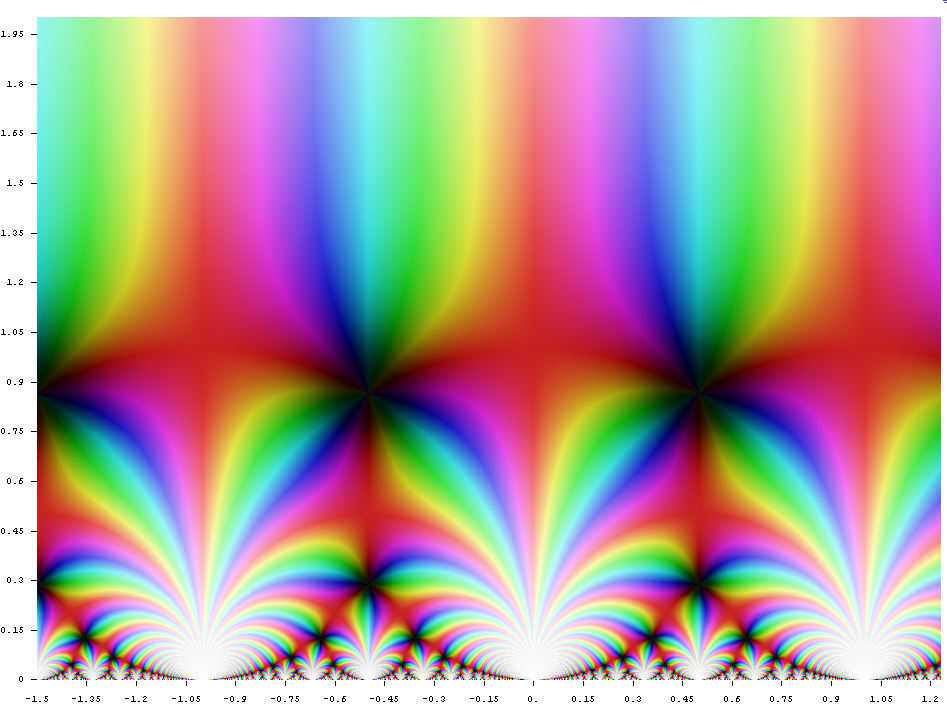We propose a new higher dimensional version of the McKay correspondence which enables us to understand the Hodge theory assigned to singular Gorenstein varieties by physicists, and so-called Higgs bundles.

Hodge theory can be extended to cohomology with coefficients in nonabelian groups between flat vector bundles which, by the Riemann-Hilbert correspondence, are the same as local systems (Hodge Theory in String Theory)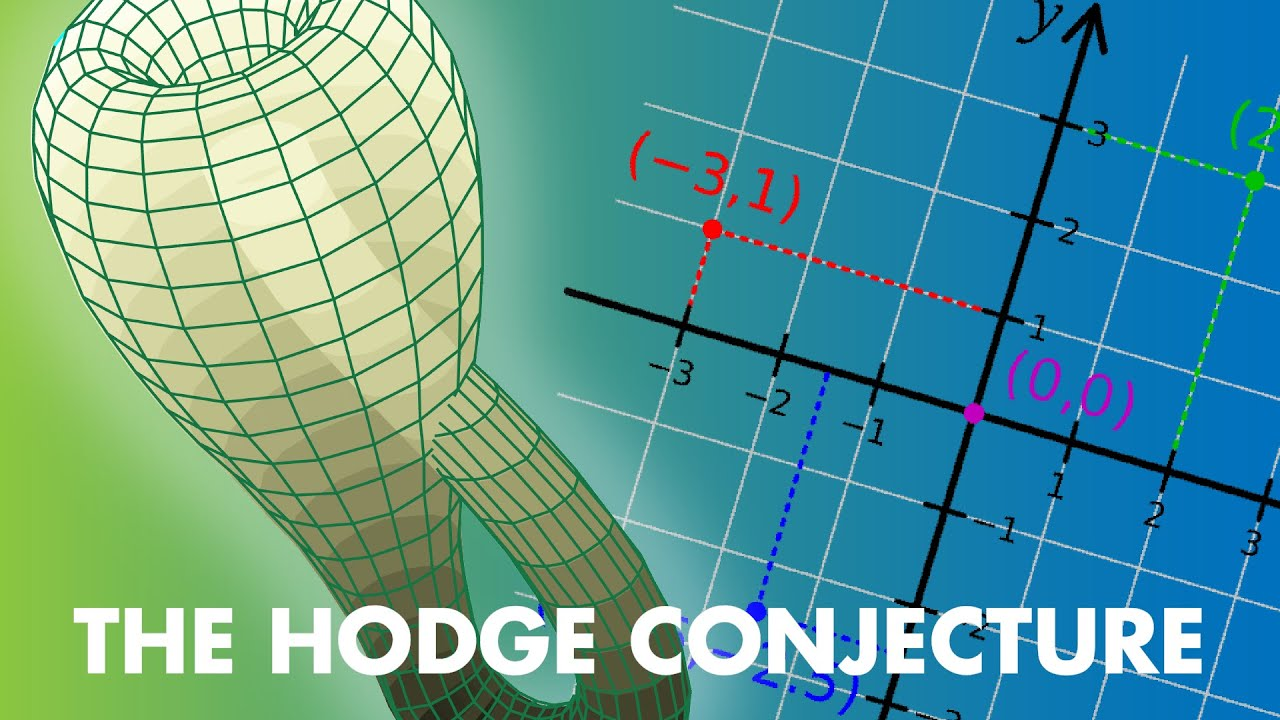Our results lead to the conjecture that string theory indicates the existence of some new cohomology theory for algebraic varieties with Gorenstein singularities.